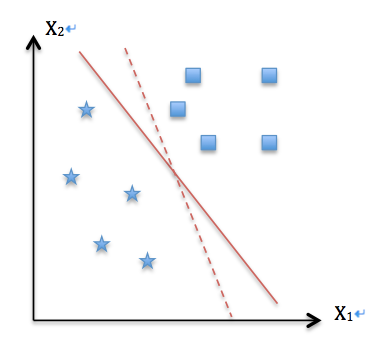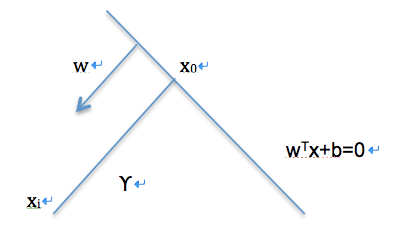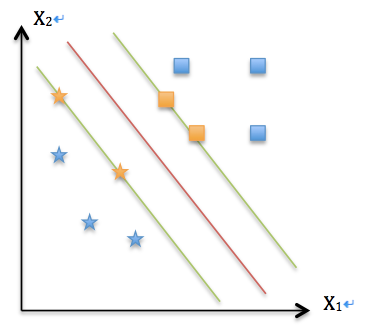SVM是一种二类分类模型，其求解目标在于确定一个分类的超平面，以最大化特征空间上的间隔。分类超平面的确定只取决于少数的样本信息，这些关键的样本被称之为支持向量Support Vector，这也是SVM–支持向量机名称的由来。$\widehat{\gamma}=|w^Tx+b|=y_i(w^Tx+b)$

$\widetilde{\gamma}=\frac{|w^Tx_i+b|}{||w||}=\frac{y_i(w^Tx+b)}{||w||}=\frac{\widehat{\gamma}}{||w||}$

（$||w||$是向量$w$的范数，是对$w$长度的一种度量）。于是我们得到了函数间隔和几何间隔的数值关系。$\max \widetilde{\gamma}=\widehat{\gamma}/||w||, s.t. y_i(w^Tx+b)>=\widehat{\gamma}, i=1, …, n$

$\max \widetilde{\gamma}=1/||w||, s.t. y_i(w^Tx+b)>= 1, i=1, …, n$